blog

# Using the Kelly Criterion in Investing

#### Options Trading 101 - The Ultimate Beginners Guide To Options

As Seen On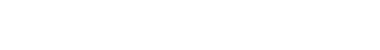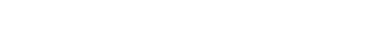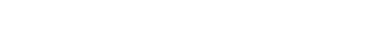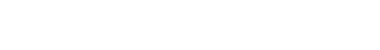by Gavin in BlogJohn Kelly developed his formula whilst working at AT&T’s Bell Laboratory when attempting to solve their long-distance signal noise issues.

It was first repurposed as a formula to identify an optimal betting system in horse racing. It allowed gamblers to increase the size of their winnings over a long period of time.

In current times, the Kelly Criterion is used in a similar purpose for investors around the world.

Two simple components make up the Kelly Criterion, the first is the probability that a trade you make will return positive winnings – and the second is the win/loss ratio.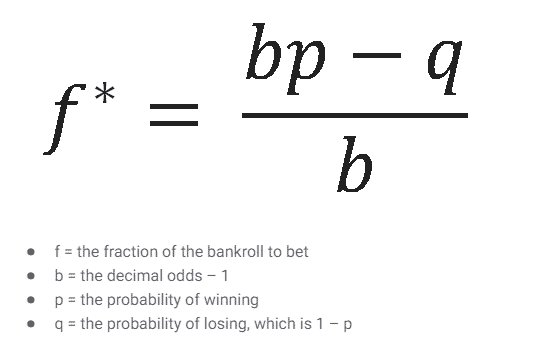A win/loss ratio is self-explanatory, you can calculate it by dividing the positive trade amounts by the negative trade amounts.

The two factors mentioned above are then inserted into the formula where the output is a percentage.

This percentage amount is crucial for investors as it enables them to understand what size of their portfolio should be given to each investment.

Here is a step by step introduction on how to use the criterion for your portfolio:

1. Pull up your last 40-60 trades, you may need to ask your broker for this information, or you can refer to past tax returns which should outline the trades if done correctly. It is important to note that the formula assumes that you invest in a similar manner now than you did in the past.

2. Calculate ‘W’, which is the winning probability. Like previously mentioned, do this by dividing the number of trades which have yielded a positive return by the number of trades you have completed overall, regardless of their outcome. If you get a number above 0.50 that is excellent

3. The win-loss ratio in the formula is represented as ‘R’. To calculate find the average gain on your positive trades and vice versa for your negative trades. Divide them together and you should be left with a number either over 1 or under 1. A result above 1 indicates that your average gains outweigh your average losses.

4. Insert these numbers into the equation and record the output ‘K’ as a percentage.

The percentage you receive as the output should indicate to you how much of your capital should be invested in each of the positions you take.

For example, if the percentage is 0.08, then you should invest an 8% position in each of the investments in your portfolio.

Essentially, the equation produces a rough guide on how much diversification should be included within your investments.

There is no system of money management that can be 100% reliable, and whilst the Kelly Criterion outlines a clear result it assumes that the trader is maintaining the same trading pattern over an extended period of time which is somewhat unrealistic.

With everything in the investment market – it is important to do your own research and not rely purely on an equation to determine the size of your portfolio.